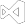# Excel to SQL and DataGrid via DataTableExcel to SQL and DataGrid via DataTable
``````using IronXL;
using System.Data

//Open any Excel document.

// Work with a single WorkSheet.  Can also use workbook.DefaultWorkSheet
WorkSheet sheet = workbook.GetWorkSheet("sheet1");

//Convert the worksheet to System.Data.DataTable
//Boolean parameter sets the first row as column names of your table.
Syste.Data.DataTable dataTable = sheet.ToDataTable(true);

//The DataTable can populate a DataGrid for example.

//Enumerate by rows or columns first at your preference
foreach (DataRow row in dataTable.Rows)
{
for (int i = 0; i < dataTable.Columns.Count; i++)
{
Console.Write(row[i]);
}
}``````
``````Imports IronXL
Imports System.Data WorkBook workbook = WorkBook.Load("test.xls")
'Open any Excel document.

' Work with a single WorkSheet.  Can also use workbook.DefaultWorkSheet
Private sheet As WorkSheet = workbook.GetWorkSheet("sheet1")

'Convert the worksheet to System.Data.DataTable
'Boolean parameter sets the first row as column names of your table.
Private dataTable As Syste.Data.DataTable = sheet.ToDataTable(True)

'The DataTable can populate a DataGrid for example.

'Enumerate by rows or columns first at your preference
For Each row As DataRow In dataTable.Rows
For i As Integer = 0 To dataTable.Columns.Count - 1
Console.Write(row(i))
Next i
Next row``````

Convert any Excel worksheet to a `System.Data.DataTable` for full interoperability with `System.Data.SQL` or to populate a DataGrid.